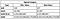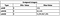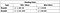## Boolean

`package mainimport (     "fmt")func main() {     var a bool = true     var b bool = false// Variables in Go are assigned 0 values unless you explicitly set them like above. The 0 value for a boolean is false     var c bool      fmt.Printf("%v, %T\n", a, a)     fmt.Printf("%v, %T\n", b, b)     fmt.Printf("%v, %T\n", c, c)}Output: true, boolfalse, boolfalse, bool`
`package mainimport (     "fmt")func main() {     a := 7 == 7     b := 4 == 7     fmt.Printf("%v, %T\n", a, a)     fmt.Printf("%v, %T\n", b, b)}Output:true, boolfalse, bool`

## Numeric

`package mainimport (     "fmt")func main() {     var i int = 16     fmt.Printf("%v, %T\n", i, i)}16, int`Value ranges are approximate and provided by “Learn Go Programming — Golang Tutorial for Beginners”Value ranges are approximate and provided by “Learn Go Programming — Golang Tutorial for Beginners”Value ranges provided by “Learn Go Programming — Golang Tutorial for Beginners”
`package mainimport (     "fmt")func main() {// Integers: Example below shows type conversion     var a int = 10     var b int8 = 3     fmt.Println(a + int(b))     // Operations available to Integers        // Arithmetic          e := 7          f := 4          fmt.Println(e + f)          fmt.Println(e - f)          fmt.Println(e * f)          fmt.Println(e / f)          fmt.Println(e % f) // % is the modulo operator and shows          the remainder of integer division       // Bit Operators (watch video for further explanation -       https://www.youtube.com/watch?v=YS4e4q9oBaU) - ~ 1 hour mark          fmt.Println(e & f)          fmt.Println(e | f)          fmt.Println(e ^ f)          fmt.Println(e &^ f)       // Bit Shifting Operators (watch video for further       explanation - https://www.youtube.com/watch?v=YS4e4q9oBaU) -        ~ 1 hour mark          fmt.Println(e << 4)          fmt.Println(e >> 4)// Floating point: In the below example n = 13.7e72 is too large for a float32     var n float64 = 3.14     n = 13.7e72     n = 2.1e14     fmt.Printf("%v, %T\n", n, n)     // Operations available to Floating Point       // Arithmetic           c := 9.7          d := 4.8          fmt.Println(c + d)          fmt.Println(c - d)          fmt.Println(c * d)          fmt.Println(c / d)}Output:// Integer Type Conversion13// Integer Arithmetic1132813// Integer Bit Operators4733// Integer Bit Shifting1120// Floating Point2.1e+14, float64// Floating Point Arithmetic 14.54.899999999999999546.5599999999999952.0208333333333335`
`package mainimport (     "fmt")func main() {// Simple Example     var n complex64 = 4i     fmt.Printf("%v, %T\n", n, n)// Arithmetic Operations     a := 4 + 2i     b := 7 + 1.9i     fmt.Println(a + b)     fmt.Println(a - b)     fmt.Println(a * b)     fmt.Println(a / b)// Splitting apart Real and Imaginary Parts using built-in functions     var c complex64 = 2 + 4i     fmt.Printf("%v, %T\n", real(c), real(c))     fmt.Printf("%v, %T\n", imag(c), imag(c))// Make a complex number using complex function. First input in complex function is the real number and the second is the imaginary     var m complex128 = complex(2, 4)     fmt.Printf("%v, %T\n", m, m)}Output:// Simple Example(0+4i), complex64// Arithmetic Operations(11+3.9i)(-3+0.10000000000000009i)(24.2+21.6i)(0.6044478236076791+0.12164987644934423i)// Splitting apart Real and Imaginary Parts2, float324, float32// Using Complex function to make a complex number(2+4i), complex128`

## Text

`package mainimport (     "fmt")func main() {     str := "Hello, Medium!"     fmt.Printf("%v, %T\n", str, str)}Output:Hello, Medium!, string`
`package mainimport (     "fmt")func main() {     str1 := "This is a"     str2 := " "     str3 := "string"     fmt.Printf("%v\n", str1+str2+str3)}Output:This is a string`

Written by